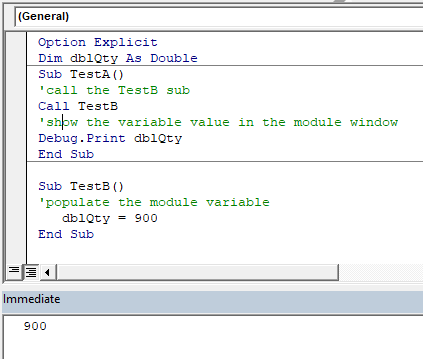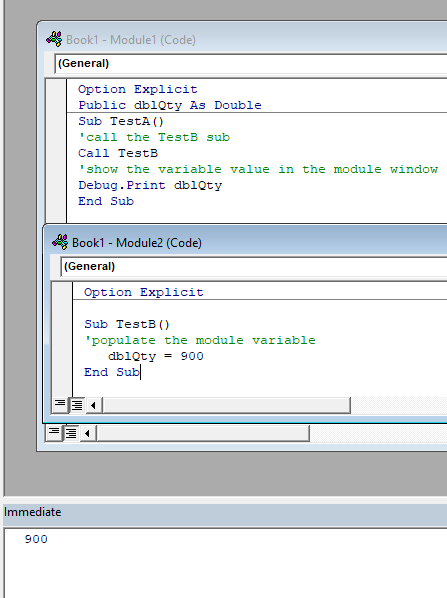# VBA – Return a Value from a Sub Procedure

This tutorial will demonstrate how to return a value from a sub procedure in VBA.

A sub procedure is not designed to return a value – that is what a function procedure is used for. However, there may be a time where you are running some code in a sub procedure where you need to get a value from that sub procedure. The way to do this is to use Module or Global Variables or to use a Sub Procedure to populate an Excel sheet.

## Using Variables to Return a Value

You can declare variables at the module-level. When doing so, those variables can be used anywhere within the module and the variables retain their values. To declare a variable at the module-level, simply declare the variable at the top of your code module:

``````Option Explicit
Dim dblQty as Double

Sub TestA()
'call the TestB sub
Call TestB
'show the variable value in the module window
Debug.Print dblQty
End Sub``````
``````Sub TestB()
'populate the module variable
dblQty = 900
End Sub``````

To put these together, we run TestA, which in turn would call TestB and give us the value of 900 in the immediate window.This only works within the module, as the variable has been declared using the Dim statement at Module level.

### Global Variable

You can declare the variable at a global level to use it throughout your project modules.## Using a Sub Procedure to Populate an Excel Sheet

Another way to reference values calculated from a Sub Procedure, is to simply write the data to an Excel Sheet and then reference that data in another procedure.

``````Sub PopulateRange()
Range("A1") = "Product"
Range("B1") = "Quantity"
Range("C1") = "Cost"
End Sub``````

You can then reference those values in another procedure by referencing the cell values:

``````Sub RetrieveRange()
Dim Product as String, Quant as long, Cost as double

Product = Range("A1")
Quant = Range("B1")
Cost = Range("C1")
End Sub``````

Stop searching for VBA code online. Learn more about AutoMacro - A VBA Code Builder that allows beginners to code procedures from scratch with minimal coding knowledge and with many time-saving features for all users!